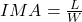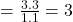## You use a wedge that has a width of 1.1 in and a slope length of 3.3 in. What is the mechanical advantage of this wedge? O A. 4.

Question

You use a wedge that has a width of 1.1 in and a slope length of 3.3 in. What
is the mechanical advantage of this wedge?
O A. 4.5
O B. 2.2
O C. 0.33
O D.3

in progress 0
5 months 2021-08-10T05:32:17+00:00 1 Answers 12 views 0

## Answers ( )

D. 3

Explanation:

First of all the mechanical advantage of a wedge is defined as the ratio of its slope length to its width.

Mathematically,where,

L= slope length, and

w= width.

Given that,

slope length, L= 3.3 inches, and

width ,W= 1.1 inches

Then,